# A Navigation Accuracy Evaluation Method for Multi-path Platform Inertial Navigation System

Space Control and Inertial Technology Research Center, Harbin Institute of Technology, Harbin 150001, China

Corresponding Author Email:
ygx@hit.edu.cn
Page:
115-121
|
DOI:
https://doi.org/10.18280/i2m.180204
10 January 2019
|
Accepted:
9 March 2019
|
Published:
30 June 2019
| Citation

OPEN ACCESS

Abstract:

The purpose of this study was to investigate estimation of the navigation accuracy of the high-precision platform inertial navigation system (PINS) rapidly. However, the prediction accuracy often plunges deeply when the model is trained by numerous flight paths. The PP-LSSVM approach was adopted to solve the problem with sparse solutions. The high-dimensional input data were dimensionally reduced by the principal component analysis (PCA); The sparsity of the model was improved by the pruning algorithm, aiming to reduce the computing load and prediction time. Thus, the proposed model is denoted as the PP-LSSVM. The results obtained in this study include the PP-LSSVM outperformed the LSSVM in prediction time by an order of magnitude, while satisfying the accuracy requirement. The results indicated that the research provides a suitable evaluation model for navigation accuracy of multi-path PINS.

Keywords:

platform inertial navigation system (PINS), navigation accuracy, principal component analysis (PCA), least squares support vector machine (LSSVM), pruning algorithm

1. Introduction

In recent years, China has made great progress on the theories and production techniques for platform inertial navigation system (PINS), laying the basis for the mass production of high-precision PINS . The PINS enjoy high reliability and a long life. However, individual PINSs may differ in navigation accuracy, as they are produced with different techniques and designed to work in different environments. The users are generally concerned about the reliability and real-time navigation accuracy of an individual system, which are critical to the operation of weapon systems and ship navigation. Therefore, it is of great practical significance to evaluate the navigation accuracy of the PINS. There are many difficulties in the application of PINS. For example, the PINS of long-term storage should be calibrated on time, but it is very laborious to calibrate the numerous inertial navigation platforms. Meanwhile, the PINS of short-term storage cannot be quickly assembled to the weapon system without real-time prediction and compensation of parameter accuracy . In addition, it is difficult for decision-makers to quickly select the PINS with a suitable accuracy range.

Much research has been done to estimate the accuracy of strapdown inertial systems and integrated navigation systems. For example, Reference  employs secondary optimization to identify the error parameters in high-precision strapdown inertial system, and thus effectively improves the navigation accuracy of hypersonic aircrafts. Reference  proposes a parameter identification and reconstruction algorithm to identify the apparent motion from accelerometer measurement containing random noise and the results fulfill self-alignment in a swinging condition and the alignment accuracy can reach the theoretical values. Reference  develops a new specific force integration algorithm to eliminate approximation error of the traditional method, simulation test demonstrates the accuracy and good overall performance. Reference [6-7] designs a geomagnetic-assisted inertial navigation algorithm based on Bayesian estimation, which estimates the position accuracy by probability estimation. Reference  puts forward a method to enhance the accuracy of the inertial navigation system when the integrated navigation system is offline. Reference  analyzes the slow maneuvering accuracy of an integrated inertial navigation system. Unlike the above studies, Reference  combines the least squares support vector machine (LSSVM) and the navigation solution model to predict the PINS accuracy, but the combined model is limited in that it only considers a single flight path.

The inertial navigation system is nonlinear and time-varying. The navigation accuracy of the PINS depends on both the error of the inertial device, and the motion form of the carrier. If the motion form is complex, the system will have a high navigation error. Typical motion forms like turning, overloading and maneuvering have their unique error-inducing features. Together, these motion forms constitute the flight path of the aircraft. The way to predict PINS navigation accuracy is to refer to both simple and complex paths, consider the similarities and differences between multiple paths, and extract the inter-path eigenvalues as the model inputs, paving the way for the accurate prediction of multiple paths. Since the volume of input data increases with the number of paths, it is necessary to identify the effective data that affect the final predicted results and eliminate the low-impact data based on system performance and inter-path features. Meanwhile, the growing data volume will suppress the computing speed and generalization ability of the LSSVM. Therefore, the LSSVM should be modified to enhance its generalization ability and reduce the computing load, such as to achieve rapid prediction without sacrificing the evaluation effect. Considering the above problems in rapid prediction of PINS accuracy, this paper proposes a dimensionality-reducing sparse LSSVM that can rapidly predict the accuracy of the PINS with multiple paths.

This paper will be divided into the following parts to introduce the navigation accuracy evaluation method for multi-path platform inertial navigation system. The first section analyses and summarizes the literature in related fields, and points out the unsolved problems. The second section designs PINS. The third section designs the extraction method of path features. The fourth section gives the evaluation method of navigation accuracy of multi-path platform inertial navigation system based on pruning LSSVM. The fifth section carries out simulation experiments, and finally, the sixth section summarizes and analyses the methods proposed in this paper.

2. Pins Design

The wander-azimuth system was selected as the basis for our PINS design, as it overcomes the abnormality of the north-pointing system at high latitudes. To obtain the PINS error, the fourth-order Runge-Kutta algorithm was introduced to solve the error model of the wander-azimuth system, which contains the differential equations of the attitude angle error, flight velocity error, and positioning error . The differential equation of the positioning error of the wander-azimuth PINS can be expressed as:

$\begin{cases} \delta \dot{L}=\frac{\sin \alpha \delta v_{x}^{w}+\cos \alpha \delta v_{y}^{w}+\left(\cos \alpha v_{x}^{w}-\sin \alpha v_{y}^{w}\right) \delta \alpha}{R_{e}+h}-\frac{\sin \alpha v_{x}^{w}+\cos \alpha v_{y}^{w}}{\left(R_{e}+h\right)^{2}} \delta h\\ \delta i=\frac{\cos \alpha \delta v_{x}^{w}-\sin \alpha \delta v_{y}^{w}-\left(\sin \alpha v_{x}^{w}+\cos \alpha v_{y}^{w}\right) \delta \alpha}{R_{e}+h}-\frac{\cos \alpha v_{x}^{w}-\sin \alpha v_{y}^{w}}{\left(R_{e}+h\right)^{2} \cos L} \delta h+\frac{\cos \alpha v_{x}^{w}-\sin \alpha v_{y}^{w}}{\left(R_{e}+h\right) \cos L} \operatorname{tg} L \delta L\\ \delta \dot{h}=\delta V_{z} \\ \delta \dot{\alpha}=\frac{\cos \alpha V_{x}^{w}-\sin \alpha V_{y}^{w}}{\left(R_{e}+h\right)^{2}} \operatorname{tg} L \delta h-\frac{\cos \alpha V_{x}^{w}-\sin \alpha V_{y}^{w}}{\left(R_{e}+h\right) \cos ^{2} L} \delta L \end{cases}$                               (1)

where, in, w is the ideal platform coordinate system of the wander azimuth system; α is the wander azimuth angle; $v_{x}, v_{y}, v_{z}$ are respectively the east velocity, north velocity and upward velocity, $\delta v_{x}, \delta v_{y}, \delta v_{z}$  are respectively east velocity errors, north velocity errors and upward velocity errors; l and L are the longitude and the latitude respectively; $\delta L$  and $\delta l$  are respectively longitude errors and latitude errors; h is the height of the carrier; $\delta h$ is the height errors; $R_{e}$ is the earth radius. Through derivation, the cross-coupling relationships between 39 error sources and the final system error were determined. The error sources include the gyro, accelerometer.

3. Extraction of Path Features

3.1 Path features

The navigation accuracy of the PINS is both disturbed by the error of the inertial device, and greatly affected by the flight path of the carrier. Both factors should be considered before setting up the error model of the PINS. Some of the error terms are related to the path and flight time. Thus, the navigation accuracy may vary from path to path. It is necessary to introduce feature quantities to minimize the effect of path difference. The gyro and accelerometer errors are mainly affected by the following path factors, namely, heading drift, turning time, centripetal acceleration, rotational angular velocity, turning radius and turning acceleration.

Based on path difference, three types of feature quantities were created. The first type includes feature quantities related to the rotational angular velocity, such as the gyro mounting error MG. Each of the feature quantities was constructed in two steps: integrating the error parameters along the entire path, and computing the time average. The cumulative mean of each feature quantity in the first type can be expressed as:

$\mathrm{c}_{1}=\frac{1}{T} \int_{0}^{T} e_{i} d t$  (2)

where, c is a feature quantity in the first type; T is the flight time; ei=MG is the error parameter.

The second type contains feature quantities related to acceleration , such as the term of the gyro related to the acceleration of gravity $g_{m}^{1}(m=x, y, z ; n=x, y, z)$, the scale factor of the accelerometer kA. Among them, the cumulative mean of each feature quantity in the second type can be expressed as:

$\mathrm{c}_{2 i}=\frac{1}{T} \int_{0}^{T} f \cdot P_{i} d t$  (3)

where, $P_{i}=g_{m}^{1}(m=x, y, z ; n=x, y, z), k_{A}$ ; f is the acceleration acting on the gyro. The term of the gyro related to the acceleration of gravity $P_{i}=g_{m}^{1}(m=a, b ; n=a, b)$ was cited to explain the construction of the feature quantities in the second type.

Firstly, the acceleration of the gyro was multiplied by the drift error coefficient related to the acceleration of gravity g; next, the error parameters were integrated along the entire path, yielding the cumulative acceleration of the entire path; then, the time average of the integrated result was computed, resulting in the cumulative mean acceleration in the same interval. The third type involves three feature quantities related to the heading drift. Considering the effect of heading drift on the navigation system throughout the flight, these feature quantities were constructed as those in the first type. The four feature quantities were taken as the inputs to train the SVM, so the simulation data will be increased to as many as 60 dimensions.

3.2 Principal component analysis (PCA)

The PINS is nonlinear and time-varying. The navigation accuracy of the system is subjected to varied, cross-coupled impacts from different error terms. If all main errors related to PINS navigation accuracy are adopted as the inputs to the prediction model, the simulation data are 60 dimensions. If predicted by the LSSVM, the high-dimensional data will have a serious negative impact on the computing time and the prediction effect. The high dimensionality is a signal of the presence of redundant or unrelated features in the sample, which have little to do with the output values. However, it is not recommended to identify these features subjectively, as subjective selection may wrongly remove some important factors and lower the prediction accuracy.

Considering the importance of input selection to PINS navigation accuracy, the PCA was adopted to reduce the data dimensionality , i.e. removing the redundant feature quantities with little impact on the outputs and retaining those directly affecting the position error, velocity error and attitude angle error in PINS outputs. The purpose is to provide the prediction model with proper inputs, and shorten the computing time for prediction.

The PCA is a desirable input optimization method, capable of extracting nonlinear feature information, and removing redundant, unrelated features. Below is a mathematical explanation of this method.

Let $X=\left\{x_{m} \in R^{q} | m=1,2, \dots, N\right\}$ be the sample set. By the nonlinear function $\varphi : R^{q} \rightarrow F$, the sample xm can be mapped into the data φ(xm) in the high-dimensional feature space (F space). Then, the covariance matrix can be expressed as:

$C^{F}=\frac{1}{N} \sum_{m=1}^{N} \varphi\left(x_{m}\right) \varphi\left(x_{m}\right)^{\mathrm{T}}$  (4)

where, φ(xm), m=1,2,…, N has a zero-mean. Then, the kernel function matrix K can be defined, such that Kmg=K(xm,xg),m,g=1,2,…,N. The inner product operation of the F space can be converted into an input space operation, and K(xm,xg) is a kernel function that satisfies the Mercer’s condition. If the mean of φ(xm) is non-zero, the K can be transformed into a centralized matrix $\overline{K}$ as follows:

$\overline{K}=K-A_{N} K-K A_{N}+A_{N} K A_{N}$  (5)

where, AN is a N-order matrix; am,g=1/N, m,g=1,2,…N.

The features of $\overline{K}$ can be decomposed as:

$\lambda \beta=\overline{K} \beta$  (6)

where, $\lambda=\left[\lambda_{1}, \lambda_{2}, \ldots, \lambda_{N}\right]$ are the eigenvalues of $\overline{K}$ ; $\beta=\left[\beta_{1}, \beta_{2}, \ldots, \beta_{N}\right]$ are the eigenvectors corresponding to the eigenvalues. Thus, the eigenvector of CF can be obtained as $v=\sum_{i=m}^{N}\left(\frac{\beta_{m}}{\sqrt{\lambda_{m}}}\right) \Phi\left(x_{m}\right)$. Then, the r-th nonlinear principal component can be described as:

$s_{r}=v_{r} \Phi\left(x_{m}\right)=\sum_{i=m}^{N}\left(\beta_{m, r} / \sqrt{\lambda_{m, r}}\right) K\left(x_{m}, x_{g}\right)$   (7)

The cumulative contribution rate of each eigenvalue can be calculated as:

$\sum_{i=1}^{m} \lambda_{m} / \sum_{i=1}^{n} \lambda_{n}$    (8)

where, m is the number of principal components.

4. LSSVM based on Pruning and PCA (PP-LSSVM)

4.1 LSSVM

The basic idea of LSSVM was first proposed by Suykens, Vandewalle et al. . By this algorithm, the square term is adopted as the optimization index, and the inequality constraint of the standard SVM is replaced with an equality constraint, turning the quadratic programming problem into a set of linear equations. In this way, the LSSVM achieves simple computation and rapid solving speed. Thus, this algorithm has been widely used in function estimation and approximation [15-17].

Let $\left\{\left(x_{i}, y_{i}\right)\right\}_{i=1}^{N}$ be a dataset with N training samples, $x_{i} \in R^{n}$ be the inputs and $y_{i} \in \mathrm{R}$ be the outputs. Then, the nonlinear LSSVM for regression estimation can be expressed as:

$\min \frac{1}{2}(\omega \cdot \omega)+\frac{1}{2} \gamma \sum_{\mathrm{i}=1}^{1} e_{i}^{2}$

s.t. $\left\{\begin{array}{c}{y_{i}-\omega^{\mathrm{T}} \phi\left(x_{i}\right)-b=e_{i}} \\ {i=1, \ldots, l}\end{array}\right.$  (9)

where, φ(·) is a nonlinear function that maps the input space to the high-dimensional feature space; ω is the model complexity; $e=\left[e_{1}, \ldots, e_{n}\right]^{T}$ is the empirical error; $\gamma \in R^{+}$ is the regularization parameter. With the aid of dual optimization and Lagrange function, the constrained optimization problem can be converted into an unconstrainted optimization problem:

$L=\frac{1}{2}(\omega \cdot \omega)+\frac{1}{2} \gamma \sum_{i=1}^{1} e_{i}^{2}-\sum_{i=1}^{l} \alpha_{i}\left[\omega^{\mathrm{T}} \phi\left(x_{i}\right)+b+e_{i}-y_{i}\right]$ (10)

where, αi is the Lagrange multiplier. Solving the partial differential equations for each variable of equation (10), we have:

$\left.\begin{array}{l} {\frac{\partial L}{\partial \omega}=0 \rightarrow \omega=\sum_{i=1}^{l} \alpha_{i} \phi\left(x_{i}\right)} \\ {\frac{\partial L}{\partial b}=0 \rightarrow \sum_{i=1}^{l} \alpha_{i}=0} \\ {\frac{\partial L}{\partial e_{i}}=0 \rightarrow \alpha_{i}=\gamma e_{i}} \\ {\frac{\partial L}{\partial \alpha_{i}}=0 \rightarrow \omega^{\mathrm{T}} \phi\left(x_{i}\right)+b+e_{i}-y_{i}=0} \end{array}\right\}$                     (11)

Eliminating ω and e, equation (11) can be rewritten as a matrix:

$\left[\begin{array}{cc}{0} & {1^{\mathrm{T}}} \\ {1} & {K+\gamma^{-1} I}\end{array}\right]\left[\begin{array}{l}{b} \\ {\alpha}\end{array}\right]=\left[\begin{array}{l}{0} \\ {y}\end{array}\right]$(12)

where, $y=\left[y_{1}, \ldots, y_{n}\right]^{T} ; \alpha=\left[\alpha_{1}, \ldots, \alpha_{n}\right]^{T} ; 1=\left[1_{1}, \ldots, 1_{n}\right]^{T}$; I is the identity matrix; Kij=f(xi)Tf(xi)=k(xi,xj); k(xi,xj) is the kernel function. The commonly used kernel functions include the Gaussian kernel function $k\left(x_{i}, x_{j}\right)=\exp \left(-\left\|x_{i}-x_{j}\right\|^{2} / \rho^{2}\right)$ and the polynomial kernel function $k\left(x_{i}, x_{j}\right)=\left(x_{i}^{T} \frac{x_{j}}{\mu}+1\right)^{d}$. Solving the linear equation set in equation (12), the regression function can be obtained as:

$y(x)=\omega^{\mathrm{T}} \phi(x)+b=\sum_{i=1}^{l} \alpha_{i} k\left(x_{i}, x\right)+b$  (13)

In equation (13), most to all of the components of $\alpha$ are not zero, indicating the lack of sparsity. Thus, equation (13) has numerous kernel functions. If used for generalization, this equation will cause a huge computing load.

4.2 Pruning algorithm

Under equality constraint, the LSSVM faces a lacking of sparsity. The excessive number of support vectors complicates the model structure and prolongs the prediction time. The application scope of the LSSVM is limited due to its poor generalization and slow prediction under large datasets. To overcome the limitation, the training should be accelerated to reduce the computing load, such that the algorithm is sparse enough for rapid training. Meanwhile, the generalization ability of the algorithm should be enhanced to promote the applicability.

Suykens  et al. were the first to propose a pruning algorithm that increases the sparseness and computing speed of the LSSVM. In the modelling process, the training data are trimmed continuously by a certain pruning criterion, making the solutions sparse. To implement the pruning algorithm, the small support values in the support value map should be set to zero. The algorithm can obtain sparse solutions and enhance model generalization, without solving the inverse matrix of the Hessian matrix.

In Suykens’ algorithm, the samples with a small $\left|\alpha_{i}\right|$ are deleted, such that $e_{i}=\alpha_{i} / \gamma$. This is equivalent to creating an error-insensitive domain. However, the deletion does not necessarily minimize the input error. De Kruif  et al. developed a pruning algorithm that removes the sample with the least input error from the model. The algorithm was improved by Kuh to delete the sample with the minimum $\left|\alpha_{i}\left(\gamma^{-1}-\left(A_{i, i}^{-1}\right)^{-1}\right)\right|$  . The improved pruning algorithm offers another way to input error into the model after sample removal.

According to Kuh’s pruning algorithm, the coefficient in formula (12), denoted as A, can be defined as:

$A=\left[\begin{array}{cc}{0} & {1^{\mathrm{T}}} \\ {1} & {K+\gamma^{-1} \boldsymbol{I}}\end{array}\right]$ (14)

Let P=A-1. Then, the input error after the sample training can be expressed as:

$\Delta e_{i}=\alpha_{i}\left(\frac{1}{\gamma}-\frac{1}{p(i, i)}\right)$   (15)

where, p(i,i) is the i-th diagonal element of P. If the absolute value of the input error |ei| is small, then the support vector corresponding to the error must have little impact on the model, and can be deleted first. Our pruning algorithm was trained in Figure 1. To be specific, Train the LSSVM based on the sample set and then calculate the kernel matrix K. Calculate matrix A according to equation (14). Compute the output error ei induced by each support vector of the current model by equation (15): ei (i=1,2,…,Nsυ). Sort the absolute values of the output errors in ascending order, and create a new support vector set SV containing the support vectors corresponding to the output errors; Delete the top 5 % of the support vectors from the SV, and retrain the model with the remaining support vectors. Go to Step (1) if the performance index does not decline; Otherwise, terminate the training and obtain the sparse LSSVM.

## figure_1.png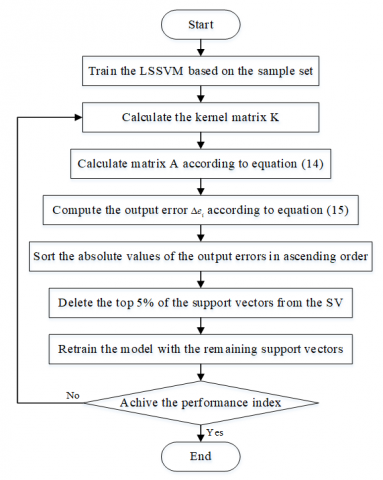Figure 1. The flowchart of training pruning algorithm in LSSVM

5. Simulation Experiment

The model proposed in this paper applies to multi-path systems. Hence, 6 paths were created on a path generator for simulation experiment. These paths have the same flight time (50min), sampling frequency (10Hz), initial velocity (235 m/s) and initial position, but differ in initial heading angle, turning time and turning radius. The error parameters of the gyro and the accelerometer were calculated by the established PINS error model (Table 1), after the required error parameter values were produced through Monte-Carlo random sampling.

Table 1. Error parameter setting

 Error source value Gyroscope constant drift 0.01(°)/h scale factor error 1´10-5 error parameter related to the accelerated velocity 0.2°/h/g the square term of the specific force input 0.05°/h/g2 installment error 1’ accelerometer constant bias 50μg scale factor error 5´10-5 installation error 1’

The nonlinear differential error model was solved by the Runge-Kutta algorithm. The solving process of the navigation system was driven by the actual path data from the path generator. The results were saved for further analysis. The 10 error items in the results were combined with the 49 terms into the experimental dataset. The 49 terms include the 41 error terms (e.g. the gyro error, the accelerometer error and the heading error) and the 8 feature quantities created from flight paths in Section 3. The dataset was denoted as the input dataset xi. As a unitary linear regression model, the LSSVM outputs a one-dimensional result. Therefore, one of the ten error terms in the results was taken as the output data of the sample set, such as longitude error, latitude error, velocity error, position error, etc.

Here, the northbound position errors are treated as the outputs of the sample set and collectively referred to as the output dataset yi. Then, the dataset (xi,yi) was divided into a training dataset (xT,yT) and a test dataset (xC,yC). The former contains 80 % of the data in (xi,yi), while the latter covers the other 20 %. The error model was employed to solve the six paths, 200 times per path, yielding 1,200 sample points. Among them, 960 sample points were selected as the training data (xT,yT) and the remaining 240 sample points as the test data (xC,yC).

After the training and test data were constructed, the input data had a staggering number of 49 dimensions. As a result, the dimensions of the input parameters were compressed effectively by the PCA, and the mean absolute percentage error (MAPE) between the predicted and actual values was computed. The MAPE reflects the effect of dimension compression on prediction accuracy. This index can be calculated as:

$\operatorname{MAPE}=\sum_{i=1}^{n}\left|\frac{\hat{y}(i)-y(i)}{y(i)}\right| * \frac{100}{n}$ (16)

where, $\hat{y}(i)$ is the value predicted by the model; y(i) is the actual value; n is the number of samples. As shown in Figure 2, the MAPE basically remained in 0.1~0.4 when the input dimensions were compressed from 60 to 26, and gradually increased to 103 with further growth in the input dimensions. Thus, 26 is the most suitable number of dimensions of the input data. Using 26-dimensional input data, the model can make accurate predictions with low-dimensional inputs and require less computing load in the training process.

## figure_2.png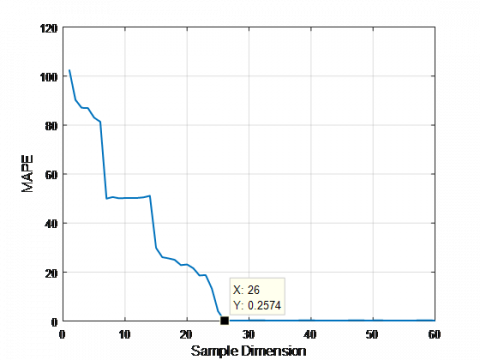Figure 2. Average absolute percentage error of input data based on PCA compression

After being dimensionally-reduced, the input data of the LSSVM were trimmed by the above-mentioned pruning algorithm. Here, the pruning performance is evaluated by the root mean square error (RMSE):

$\mathrm{RMSE}=\sqrt{\frac{1}{n} \sum_{i=1}^{n}|\hat{y}(i)-y(i)|^{2}}$    (17)

## figure_3.pngFigure 3. Root mean square error of northbound position corresponding to different pruning rates

## figure_4a.png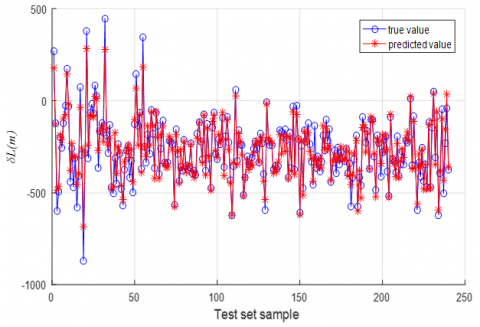## figure_4b.png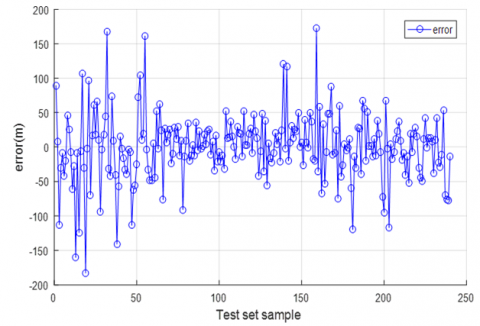Figure 4. (a) The actual value and predicted value of the North position error of the test set based on least squares support vector machine; (b) The difference between the northward actual value and the predicted value of the test set based on the least squares support vector machine

Figure 3 shows that the RMSE of the corresponding northbound position changed slightly at the initial growth in pruning rate. However, this error started to soar after the rate surpassed 60 %. Therefore, the pruning rate was set to 60 % for the data training and testing of the LSSVM. Since it is modified by pruning and PCA, the proposed LSSVM is denoted as the PP-LSSVM.

Figure 4(a) compares the northbound position error curve predicted by the LSSVM and the actual error curve, and Figure 4(b) presents the curve of the differences between the northbound position errors predicted by the LSSVM and the actual errors. It can be seen from Figure 4(b) that the most differences concentrated at about 50m and only a few fell between 100 and 180m. Obviously, the prediction results cannot satisfy the requirements of high-precision inertial navigation systems running continuously for 50mins.

## figure_5a.png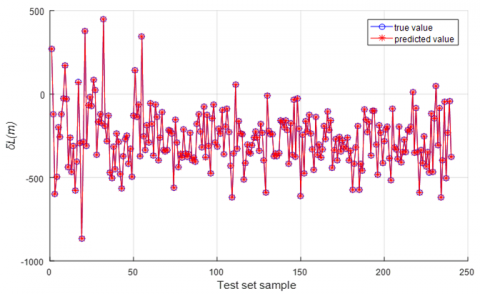## figure_5b.pngFigure 5. (a) Comparisons between predicted and actual values of northward position error based on PRLSSVM test set; (b) Northbound position error difference based on PRLSSVM error test.

Figure 5(a) compares the northbound position error curve predicted by the PP-LSSVM and the actual error curve, and Figure 5(b) presents the curve of the differences between the northbound position errors predicted by the PP-LSSVM and the actual errors. It is clear that all differences were within 1m. This outcome satisfies the application demand and the prediction requirements.

Figures 6 displays the MAPE between the northbound position error predicted by the LSSVM and the actual error, and Figure 7 shows that between the northbound position error predicted by the PP-LSSVM and the actual error. It can be seen that the PP-LSSVM, with a smaller-than-one MAPE, is more suitable than the LSSVM for the accuracy prediction of multi-path model.

## figure_6.png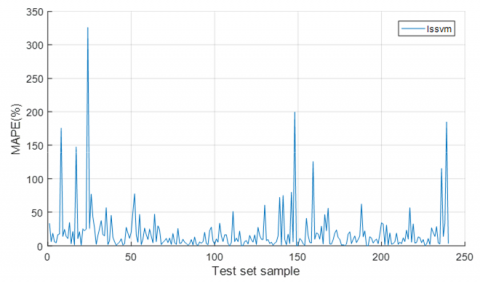Figure 6. Absolute percentage error between actual and predicted values of northbound position error based on LSSVM test set

## figure_7.png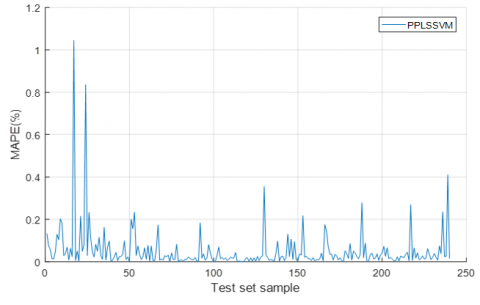Figure 7. Absolute percentage error between actual and predicted values of northbound position error based on LSSVM test set

Table 2. The set of data results (1200 sample points for calculating six tracks)

 Algorithm Sample set Support vector number RMSE Prediction time (s) LSSVM 1200 1200 48.412 68.2 PLSSVM 1200 1200 1.683 25.6 PPLSSVM 1200 480 1.777 6.9

As shown in Table 2, the LSSVM contained 1,200 support vectors, when trained by 1,200 sample points. In this case, this model consumed 68.2s to predict the northbound position, and the RMSE of the predicted positions stood at 48.412. After the input data were dimensionally reduced by the PCA, the LSSVM can be denoted as the P-LSSVM, with P standing for the PCA. For this model, the number of support vectors remained as 1,200, the prediction time was 25.6s and the RMSE was 1.683. Thus, the PCA dimensionality reduction can greatly enhance the prediction accuracy, and slightly improve the prediction time. As for the PP-LSSVM, the number of support vectors was reduced to 480 and the prediction time dropped to 6.9s, despite a slight increase in the RMSE. Overall, the PP-LSSVM outperformed the LSSVM in prediction time by an order of magnitude, thanks to the good solution sparsity and generalization ability of the PP-LSSVM. The excellent prediction time satisfy the prediction demand for multi-path PINS.

6. Conclusions

This paper extracts the path features according to the heading effect of the multi-path PINS, and develops an improved LSSVM based on the PCA and the pruning algorithm (PP-LSSVM) for the prediction of PINS navigation accuracy. The PCA and pruning effectively reduced the computing load and enhanced the generalization ability of the prediction model, and ensured the sparsity of model solutions. The simulation experiment proved that the proposed PP-LSSVM achieved high accuracy and consumed a short time in the accuracy prediction of multi-path PINS. The real-time, accurate prediction enables the rapid decision-making in the PINS.

References

 Dang, J. (2011). Implementation of high precision platform inertial navigation system. Journal of Missiles and Guidance, 31(3): 59-62. https://doi.org/10.3969/j.issn.1673-9728.2011.03.017

 Qian, C., Zhang, Z.J., Li, D.W. (2017). On-line reliability assessment of platform inertial navigation system. Journal of Aviation, 38(9): 321259-1-321259-7. https://doi.org/10.7527/S1000-6893.2017.321159

 Guo, W.L., Xian, Y., Zhang, D.Q., Li, B. (2018). Quadratic optimization identification research on error parameters of SINS for hypersonic vehicle. Control and Decision, 34(6): 1-9. https://doi.org/10.13195/j.kzyjc.2018.0604

 Lin, Y.R., Zhang, W., Xiong, J.Q. (2015). Specific force integration algorithm with high accuracy for strapdown inertial navigation system. Aerospace Science and Technology, 42: 25-30. https://doi.org/ 10.1016/j.ast.2015.01.001

 Liu, X.X., Zhao, Y., Liu, X.J., Yang, Y., Song, Q., Liu, Z.P. (2014). An improved self-alignment method for strapdown inertial navigation system based on gravitational apparent motion and dual-vector. Review of Scientific Instruments, 85(12): 3836-3846. https://doi.org/10.1063/1.4903196

 Liu, Y.F., Zheng, P.C. (2017). A Bayesian estimation-based algorithm for geomagnetic aided inertial navigation. Acta Scientiarum Naturalium Universitatis Pekinensis, 53(5): 873-880. https://doi.org/10.13209/j.0479-8023.2017.016

 Chen, D.D. (2017). Studies on SINS calibration performance quantitative evaluation approach based on bayesian smoothing. Harbin Engineering University, 50-120. https://doi.org/CNKI:CDMD:1.1018.044385

 Filyashkin, N.K., Yatskivsky, V.S. (2013). Prediction of inertial navigation system error dynamics in INS/GPS system. Actual Problems of Unmanned Air Vehicles Developments Proceedings (APUAVD), 2013 IEEE 2nd International Conference. https://doi.org/10.1109/APUAVD.2013.6705327

 Hadi, N., Hamed, M. (2017). Accuracy analysis of an integrated inertial navigation system in slow maneuvers. Navigation, 64(2): 197-211. https://doi.org/10.1002/navi.195

 Dang, H.T., Du, Z.L., Wang, C.H. (2014). A practical combination forecasting evaluation method for comprehensive performance of platform inertial navigation system. Chinese Journal of Inertial Technology, 22(1): 9-13. https://doi.org/10.13695/j.cnki.12-1222/o3.2014.01.003

 Wang, F.L., Wen, X.L., Wang, D.X. (2012). The error analysis of gyroscope drifs to the rate azimuth platform system. Applied Mechanics and Materials, 6: 155-156. https://doi.org/10.4028/www.scientific.net/AMM.155-156.555

 Xu, Z.Y., Yi, G.X. (2019). Acceleration drift mechanism analysis and compensation for hemispherical resonant gyro based on dynamics. Microsystem Technologies, 1-11. https://doi.org/10.1007/s00542-019-04327-0.

 de Araújo Padilha, C.E., de Santana Souza, D.F., de Araújo, J.V., Júnior, S.D.O., Macedo, G., Santos, E. (2016). Baker’s yeast invertase purification using Aqueous Two Phase System-Modeling and optimization with PCA/LS-SVM. Food and Bioproducts Processing, 101: S0960308516301559. https://doi.org/10.1016/j.fbp.2016.11.004

 Suykens, J.K., Vandewalle, J. (1999). Training multilayer perceptron classifiers based on a modified support vector method. IEEE Transactions on Neural Network, 10(4): 907-911. https://doi.org/10.1109/72.774254

 Razavi, R., Kardani, N., Ghanbari, A., Lariche, M.J., Baghban, A. (2018). Utilization of LSSVM algorithm for estimating synthetic natural gas density. Petroleum Science and Technology, 36(11): 1-6. https://doi.org/10.1080/10916466.2018.1447954

 Wang, L.Y., Liu, Z., Chen, C.L.P., Zhang, Y., Lee, S. (2012). Support vector machine based optimal control for minimizing energy consumption of biped walking motions. International Journal of Precision Engineering & Manufacturing, 13(11): 1975-1981. https://doi.org/10.1007/s12541-012-0260-7

 Barati-Harooni, A., Najafi-Marghmaleki, A., Arabloo, M., Mohammadi, A.H. (2016). An accurate CSA-LSSVM model for estimation of densities of ionic liquids. Journal of Molecular Liquids, 224(A): 954–964. https://doi.org/ 10.1016/j.molliq.2016.10.027

 Suykens, J.A.K., Lukas, L., Vandewalle, J. (2002). Sparse approximation using least squares support vector machines. IEEE International Symposium on Circuits & Systems. https://doi.org/10.1109/ISCAS.2000.856439

 Kruif, B.J., Vries, T.J. (2003); Pruning error minimization in least squares support vector machines. Neural Networks IEEE Transactions, 14(3): 696-702. https://doi.org/10.1109/TNN.2003.810597

 Kuh, A., Wilde, P.D. (2007). Comments on \"pruning error minimization in least squares support vector machines. IEEE Transactions on Neural Networks, 18(2): 606-609. https://doi.org/10.1109/TNN.2007.891590